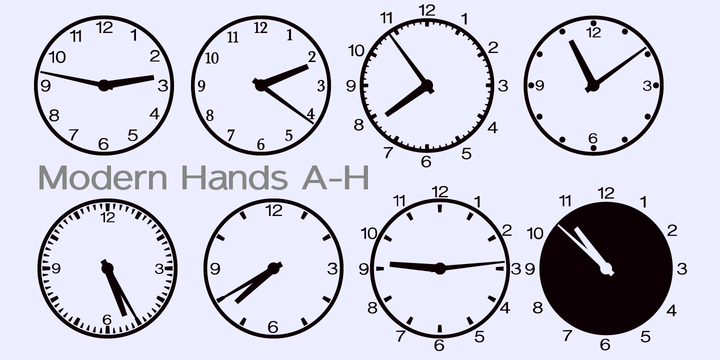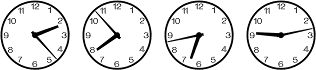1000Fonts.com

# Time Exactly™

Home / Fonts / Time / Time Exactly™### What is the Time Exactly™ font?

A lot of fonts that will give you the time as a picture of a clock face, with the exact time - to the minute, as well as letters, numbers and punctuation, (as seen in the images in the gallery). It’s simplicity itself - just type in the four numbers of any time from 0000 to 2359 and it'll give you that clock face, in the style of your choice, (and there’s plenty of choice too, 60 different styles). If you want to show the time as numerals, put a colon in as you usually see, something like 09:33 or 18:12. Leave the colon out and you'll get the clock face. Download the Instructions PDF which is in the Gallery of graphics for more details (it’s very simple to follow). More… You'll need to enable Standard Ligatures for these clock faces to show. In case you're wondering, the letters in this font are based on our Sanstone font. The naming convention is as follows: —————————————————— Mod = Modern Hands on the clockface (with various styles A to M) Ant = Antique (ornate) Hands on the clockface (with various styles A to M) Roman = Roman Numerals used, with antique style hands (A - F) Plain = No numbers on the clockface (A - H) Box = The clockface is in a square-ish box (A - E) Open = an Open style of clock face (A - D) Wide = a Wide rim to the clock (A - I) Yin and Yang = you've probably guessed already what these have on the clock face

### Time Exactly™ Font families

The Time Exactly™ includes the following font families:
• Time Exactly Mod A
• Time Exactly Mod B
• Time Exactly Mod C
• Time Exactly Mod D
• Time Exactly Mod E
• Time Exactly Mod F
• Time Exactly Mod G
• Time Exactly Mod H
• Time Exactly Mod I
• Time Exactly Mod J
• Time Exactly Mod K
• Time Exactly Mod L
• Time Exactly Mod M
• Time Exactly Ant A
• Time Exactly Ant B
• Time Exactly Ant C
• Time Exactly Ant D
• Time Exactly Ant E
• Time Exactly Ant F
• Time Exactly Ant G
• Time Exactly Ant H
• Time Exactly Ant I
• Time Exactly Ant J
• Time Exactly Ant K
• Time Exactly Ant L
• Time Exactly Ant M
• Time Exactly Roman A
• Time Exactly Roman B
• Time Exactly Roman C
• Time Exactly Roman D
• Time Exactly Roman E
• Time Exactly Roman F
• Time Exactly Plain A
• Time Exactly Plain B
• Time Exactly Plain C
• Time Exactly Plain D
• Time Exactly Plain E
• Time Exactly Plain F
• Time Exactly Plain G
• Time Exactly Plain H
• Time Exactly Box A
• Time Exactly Box B
• Time Exactly Box C
• Time Exactly Box D
• Time Exactly Box E
• Time Exactly Open A
• Time Exactly Open B
• Time Exactly Open C
• Time Exactly Open D
• Time Exactly Wide A
• Time Exactly Wide B
• Time Exactly Wide C
• Time Exactly Wide D
• Time Exactly Wide E
• Time Exactly Wide F
• Time Exactly Wide G
• Time Exactly Wide H
• Time Exactly Wide I
• Time Exactly Yin
• Time Exactly Yang

### Time Exactly™ Preview

Here is a preview of how Time Exactly™ will look. For more previews using your own text as an example, click here.It is very unlikely you'll be able to find it for free, you risk getting viruses on your computer, and even if you do find it please remember that it's illegal to use it if you didn't pay for it!

If you really want Time Exactly™ then click here to visit the download and purchase page on MyFonts to get it with the proper license. The designer and publisher deserves to be paid for their work. :)

# Similar Fonts To Time Exactly™

Font Name: Time Exactly™

Design Date: 2016

Designer(s): ?

Publisher: Aah Yes